# Bernoulli theorem

(diff) ← Older revision | Latest revision (diff) | Newer revision → (diff)

The (historically) original form of the (weak) law of large numbers. The theorem appeared in the fourth part of Jacob Bernoulli's book Ars conjectandi (The art of conjecturing). This part may be considered as the first serious study ever of probability theory. The book was published in 1713 by N. Bernoulli (a nephew of Jacob Bernoulli). The theorem deals with sequences of independent trials, in each one of which the probability of occurrence of some event ( "success" ) is. Letbe the number of trials and letbe the random variable equal to the number of successful events. The Bernoulli theorem states that, whatever the value of the positive numbersand, the probability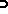of the inequality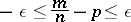will be higher than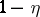for all sufficiently large(). The proof of this theorem, which was given by Bernoulli and which was exclusively based on a study of the decrease of probabilities in the binomial distribution as one moves away from the most probable value, was accompanied by an inequality which made it possible to point out a certain bound for the given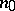ifandwere given. Thus, it was found by Bernoulli that if, the probability of the inequality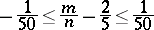will be higher than 0.999 if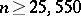. By introducing a slight improvement in the original reasoning of Bernoulli, it is possible to conclude that it is sufficient to select a value ofobeying the condition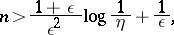which gives in turn, for the probability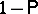of the inequality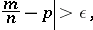an estimate of the form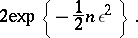The condition obtained for the above example is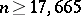(more sophisticated estimates show that it is sufficient to take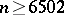; one may note, for the sake of comparison, that the de Moivre–Laplace theorem yields 6498 as the approximate value of). Other estimates formay be obtained using the Bernstein inequality and its analogues. See also Binomial distribution.

How to Cite This Entry:
Bernoulli theorem. Encyclopedia of Mathematics. URL: http://encyclopediaofmath.org/index.php?title=Bernoulli_theorem&oldid=13311
This article was adapted from an original article by Yu.V. Prokhorov (originator), which appeared in Encyclopedia of Mathematics - ISBN 1402006098. See original article